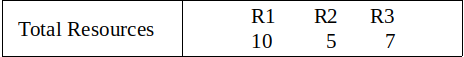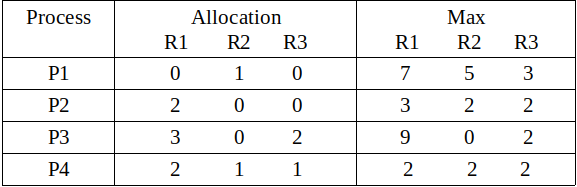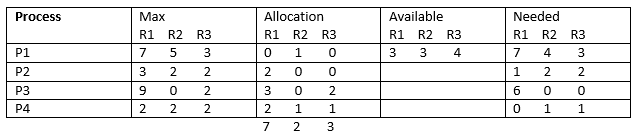Open in App
Not now

# Banker’s Algorithm in Operating System

• Difficulty Level : Medium
• Last Updated : 01 Aug, 2022

Prerequisite – Resource Allocation Graph (RAG), Banker’s Algorithm, Program for Banker’s Algorithm
Banker’s Algorithm is a resource allocation and deadlock avoidance algorithm. This algorithm test for safety simulating the allocation for predetermined maximum possible amounts of all resources, then makes an “s-state” check to test for possible activities, before deciding whether allocation should be allowed to continue.

In simple terms, it checks if allocation of any resource will lead to deadlock or not, OR is it safe to allocate a resource to a process and if not then resource is not allocated to that process. Determining a safe sequence(even if there is only 1) will assure that system will not go into deadlock.

Banker’s algorithm is generally used to find if a safe sequence exist or not. But here we will determine the total number of safe sequences and print all safe sequences.

The data structure used are:

• Available vector
• Max Matrix
• Allocation Matrix
• Need Matrix

Example:
Input:```Output: Safe sequences are:
P2--> P4--> P1--> P3
P2--> P4--> P3--> P1
P4--> P2--> P1--> P3
P4--> P2--> P3--> P1

There are total 4 safe-sequences ```

Explanation:

Total resources are R1 = 10, R2 = 5, R3 = 7 and allocated resources are R1 = (0+2+3+2 =) 7, R2 = (1+0+0+1 =) 2, R3 = (0+0+2+1 =) 3. Therefore, remaining resources are R1 = (10 – 7 =) 3, R2 = (5 – 2 =) 3, R3 = (7 – 3 =) 4.
Remaining available = Total resources – allocated resources
and
Remaining need = max – allocatedSo, we can start from either P2 or P4. We can not satisfy remaining need from available resources of either P1 or P3 in first or second attempt step of Banker’s algorithm. There are only four possible safe sequences.

```These are :
P2--> P4--> P1--> P3
P2--> P4--> P3--> P1
P4--> P2--> P1--> P3
P4--> P2--> P3--> P1```

Implementation:

## C++

 `// C++ Program to Print all possible safe sequences using banker's algorithm` `#include ` `#include ` `#include ` `// total number of process` `#define P 4` `// total number of resources` `#define R 3`   `// total safe-sequences` `int` `total = 0;`   `using` `namespace` `std;`   `// function to check if process` `// can be allocated or not` `bool` `is_available(``int` `process_id, ``int` `allocated[][R],` `                  ``int` `max[][R], ``int` `need[][R], ``int` `available[])` `{`   `    ``bool` `flag = ``true``;`   `    ``// check if all the available resources` `    ``// are less greater than need of process` `    ``for` `(``int` `i = 0; i < R; i++) {`   `        ``if` `(need[process_id][i] > available[i])` `            ``flag = ``false``;` `    ``}`   `    ``return` `flag;` `}`   `// Print all the safe-sequences` `void` `safe_sequence(``bool` `marked[], ``int` `allocated[][R], ``int` `max[][R],` `                   ``int` `need[][R], ``int` `available[], vector<``int``> safe)` `{`   `    ``for` `(``int` `i = 0; i < P; i++) {`   `        ``// check if it is not marked` `        ``// already and can be allocated` `        ``if` `(!marked[i] && is_available(i, allocated, max, need, available)) {`   `            ``// mark the process` `            ``marked[i] = ``true``;`   `            ``// increase the available` `            ``// by deallocating from process i` `            ``for` `(``int` `j = 0; j < R; j++)` `                ``available[j] += allocated[i][j];`   `            ``safe.push_back(i);` `            ``// find safe sequence by taking process i` `            ``safe_sequence(marked, allocated, max, need, available, safe);` `            ``safe.pop_back();`   `            ``// unmark the process` `            ``marked[i] = ``false``;`   `            ``// decrease the available` `            ``for` `(``int` `j = 0; j < R; j++)` `                ``available[j] -= allocated[i][j];` `        ``}` `    ``}`   `    ``// if a safe-sequence is found, display it` `    ``if` `(safe.size() == P) {`   `        ``total++;` `        ``for` `(``int` `i = 0; i < P; i++) {`   `            ``cout << ``"P"` `<< safe[i] + 1;` `            ``if` `(i != (P - 1))` `                ``cout << ``"--> "``;` `        ``}`   `        ``cout << endl;` `    ``}` `}`   `// Driver Code` `int` `main()` `{`   `    ``// allocated matrix of size P*R` `    ``int` `allocated[P][R] = { { 0, 1, 0 },` `                            ``{ 2, 0, 0 },` `                            ``{ 3, 0, 2 },` `                            ``{ 2, 1, 1 } };`   `    ``// max matrix of size P*R` `    ``int` `max[P][R] = { { 7, 5, 3 },` `                      ``{ 3, 2, 2 },` `                      ``{ 9, 0, 2 },` `                      ``{ 2, 2, 2 } };`   `    ``// Initial total resources` `    ``int` `resources[R] = { 10, 5, 7 };`   `    ``// available vector of size R` `    ``int` `available[R];`   `    ``for` `(``int` `i = 0; i < R; i++) {`   `        ``int` `sum = 0;` `        ``for` `(``int` `j = 0; j < P; j++)` `            ``sum += allocated[j][i];`   `        ``available[i] = resources[i] - sum;` `    ``}`   `    ``// safe vector for displaying a safe-sequence` `    ``vector<``int``> safe;`   `    ``// marked of size P for marking allocated process` `    ``bool` `marked[P];` `    ``memset``(marked, ``false``, ``sizeof``(marked));`   `    ``// need matrix of size P*R` `    ``int` `need[P][R];` `    ``for` `(``int` `i = 0; i < P; i++)` `        ``for` `(``int` `j = 0; j < R; j++)` `            ``need[i][j] = max[i][j] - allocated[i][j];`   `    ``cout << ``"Safe sequences are:"` `<< endl;` `    ``safe_sequence(marked, allocated, max, need, available, safe);`   `    ``cout << ``"\nThere are total "` `<< total << ``" safe-sequences"` `<< endl;` `    ``return` `0;` `}`

## Java

 `// Java Program to Print all possible safe ` `// sequences using banker's algorithm` `import` `java.util.*;`   `public` `class` `GFG` `{`   `    ``// total number of process` `    ``static` `int` `P = ``4``;` `    `  `    ``// total number of resources` `    ``static` `int` `R = ``3``;` `    `  `    ``// total safe-sequences` `    ``static` `int` `total = ``0``;`   `    ``// function to check if process` `    ``// can be allocated or not` `    ``static` `boolean` `is_available(``int` `process_id, ``int` `allocated[][],` `                    ``int` `max[][], ``int` `need[][], ``int` `available[]) ` `    ``{`   `        ``boolean` `flag = ``true``;`   `        ``// check if all the available resources` `        ``// are less greater than need of process` `        ``for` `(``int` `i = ``0``; i < R; i++)` `        ``{`   `            ``if` `(need[process_id][i] > available[i]) ` `            ``{` `                ``flag = ``false``;` `            ``}` `        ``}`   `        ``return` `flag;` `    ``}`   `    ``// Print all the safe-sequences` `    ``static` `void` `safe_sequence(``boolean` `marked[], ``int` `allocated[][], ``int` `max[][],` `                        ``int` `need[][], ``int` `available[], Vector safe) ` `    ``{`   `        ``for` `(``int` `i = ``0``; i < P; i++) ` `        ``{`   `            ``// check if it is not marked` `            ``// already and can be allocated` `            ``if` `(!marked[i] && is_available(i, allocated, max, need, available))` `            ``{`   `                ``// mark the process` `                ``marked[i] = ``true``;`   `                ``// increase the available` `                ``// by deallocating from process i` `                ``for` `(``int` `j = ``0``; j < R; j++) ` `                ``{` `                    ``available[j] += allocated[i][j];` `                ``}`   `                ``safe.add(i);` `                `  `                ``// find safe sequence by taking process i` `                ``safe_sequence(marked, allocated, max, need, available, safe);` `                ``safe.removeElementAt(safe.size() - ``1``);`   `                ``// unmark the process` `                ``marked[i] = ``false``;`   `                ``// decrease the available` `                ``for` `(``int` `j = ``0``; j < R; j++) ` `                ``{` `                    ``available[j] -= allocated[i][j];` `                ``}` `            ``}` `        ``}`   `        ``// if a safe-sequence is found, display it` `        ``if` `(safe.size() == P) ` `        ``{`   `            ``total++;` `            ``for` `(``int` `i = ``0``; i < P; i++) ` `            ``{`   `                ``System.out.print(``"P"` `+ (safe.get(i) + ``1``));` `                ``if` `(i != (P - ``1``)) ` `                ``{` `                    ``System.out.print(``"--> "``);` `                ``}` `            ``}`   `            ``System.out.println(``""``);;` `        ``}` `    ``}`   `    ``// Driver Code` `    ``public` `static` `void` `main(String[] args) ` `    ``{` `        `  `        ``// allocated matrix of size P*R` `        ``int` `allocated[][] = {{``0``, ``1``, ``0``},` `        ``{``2``, ``0``, ``0``},` `        ``{``3``, ``0``, ``2``},` `        ``{``2``, ``1``, ``1``}};`   `        ``// max matrix of size P*R` `        ``int` `max[][] = {{``7``, ``5``, ``3``},` `        ``{``3``, ``2``, ``2``},` `        ``{``9``, ``0``, ``2``},` `        ``{``2``, ``2``, ``2``}};`   `        ``// Initial total resources` `        ``int` `resources[] = {``10``, ``5``, ``7``};`   `        ``// available vector of size R` `        ``int``[] available = ``new` `int``[R];`   `        ``for` `(``int` `i = ``0``; i < R; i++)` `        ``{`   `            ``int` `sum = ``0``;` `            ``for` `(``int` `j = ``0``; j < P; j++) ` `            ``{` `                ``sum += allocated[j][i];` `            ``}`   `            ``available[i] = resources[i] - sum;` `        ``}`   `        ``// safe vector for displaying a safe-sequence` `        ``Vector safe = ``new` `Vector();`   `        ``// marked of size P for marking allocated process` `        ``boolean``[] marked = ``new` `boolean``[P];`   `        ``// need matrix of size P*R` `        ``int``[][] need = ``new` `int``[P][R];` `        ``for` `(``int` `i = ``0``; i < P; i++)` `        ``{` `            ``for` `(``int` `j = ``0``; j < R; j++)` `            ``{` `                ``need[i][j] = max[i][j] - allocated[i][j];` `            ``}` `        ``}`   `        ``System.out.println(``"Safe sequences are:"``);` `        ``safe_sequence(marked, allocated, max, need, available, safe);`   `        ``System.out.println(``"\nThere are total "` `+ total + ``" safe-sequences"``);` `    ``}` `}`   `/* This code contributed by PrinciRaj1992 */`

## Python3

 `# Python3 program to print all ` `# possible safe sequences ` `# using banker's algorithm`   `# Total number of process` `P ``=` `4`   `# Total number of resources` `R ``=` `3` ` `  `# Total safe-sequences` `total ``=` `0` ` `  `# Function to check if process` `# can be allocated or not` `def` `is_available(process_id, allocated, ` `                 ``max``, need, available):` `                     `  `    ``flag ``=` `True` ` `  `    ``# Check if all the available resources` `    ``# are less greater than need of process` `    ``for` `i ``in` `range``(R):` `        ``if` `(need[process_id][i] > available[i]):` `            ``flag ``=` `False` ` `  `    ``return` `flag`   `# Print all the safe-sequences` `def` `safe_sequence(marked, allocated, ` `                  ``max``, need, available, safe):` `    `  `    ``global` `total, P, R` `    `  `    ``for` `i ``in` `range``(P):` `        `  `        ``# Check if it is not marked` `        ``# already and can be allocated` `        ``if` `(``not` `marked[i] ``and` `            ``is_available(i, allocated, ``max``,` `                         ``need, available)):` `                             `  `            ``# mark the process` `            ``marked[i] ``=` `True` ` `  `            ``# Increase the available` `            ``# by deallocating from process i` `            ``for` `j ``in` `range``(R):` `                ``available[j] ``+``=` `allocated[i][j]` ` `  `            ``safe.append(i)` `            `  `            ``# Find safe sequence by taking process i` `            ``safe_sequence(marked, allocated, ``max``,` `                          ``need, available, safe)` `            ``safe.pop()` ` `  `            ``# unmark the process` `            ``marked[i] ``=` `False` ` `  `            ``# Decrease the available` `            ``for` `j ``in` `range``(R):` `                ``available[j] ``-``=` `allocated[i][j]` `        `  `    ``# If a safe-sequence is found, display it` `    ``if` `(``len``(safe) ``=``=` `P):` `        ``total ``+``=` `1` `        `  `        ``for` `i ``in` `range``(P):` `            ``print``(``"P"` `+` `str``(safe[i] ``+` `1``), end ``=` `'')` `           `  `            ``if` `(i !``=` `(P ``-` `1``)):` `                ``print``(``"--> "``, end ``=` `'')` `            `  `        ``print``()` ` `  `# Driver code        ` `if` `__name__``=``=``"__main__"``:` `    `  `    ``# Allocated matrix of size P*R` `    ``allocated ``=` `[ [ ``0``, ``1``, ``0` `],` `                  ``[ ``2``, ``0``, ``0` `],` `                  ``[ ``3``, ``0``, ``2` `],` `                  ``[ ``2``, ``1``, ``1` `]]` ` `  `    ``# max matrix of size P*R` `    ``max` `=` `[ [ ``7``, ``5``, ``3` `],` `            ``[ ``3``, ``2``, ``2` `],` `            ``[ ``9``, ``0``, ``2` `],` `            ``[ ``2``, ``2``, ``2` `] ]` ` `  `    ``# Initial total resources` `    ``resources ``=` `[ ``10``, ``5``, ``7` `]` ` `  `    ``# Available vector of size R` `    ``available ``=` `[``0` `for` `i ``in` `range``(R)]` `    `  `    ``for` `i ``in` `range``(R):` `        ``sum` `=` `0` `        `  `        ``for` `j ``in` `range``(P):` `            ``sum` `+``=` `allocated[j][i]` ` `  `        ``available[i] ``=` `resources[i] ``-` `sum` `    `  ` `  `    ``# Safe vector for displaying a` `    ``# safe-sequence` `    ``safe ``=` `[]` ` `  `    ``# Marked of size P for marking ` `    ``# allocated process` `    ``marked ``=` `[``False` `for` `i ``in` `range``(P)]` ` `  `    ``# Need matrix of size P*R` `    ``need ``=` `[[``0` `for` `j ``in` `range``(R)] ` `               ``for` `i ``in` `range``(P)]` `    `  `    ``for` `i ``in` `range``(P):` `        ``for` `j ``in` `range``(R):` `            ``need[i][j] ``=` `(``max``[i][j] ``-` `                    ``allocated[i][j])` `    `  `    ``print``(``"Safe sequences are:"``)` `    `  `    ``safe_sequence(marked, allocated, ``max``,` `                  ``need, available, safe)` `    `  `    ``print``(``"\nThere are total "` `+` `str``(total) ``+` `          ``" safe-sequences"``)`   `# This code is contributed by rutvik_56`

## C#

 `// C# Program to Print all possible safe ` `// sequences using banker's algorithm` `using` `System; ` `using` `System.Collections.Generic; `   `class` `GFG` `{`   `    ``// total number of process` `    ``static` `int` `P = 4;` `    `  `    ``// total number of resources` `    ``static` `int` `R = 3;` `    `  `    ``// total safe-sequences` `    ``static` `int` `total = 0;`   `    ``// function to check if process` `    ``// can be allocated or not` `    ``static` `Boolean is_available(``int` `process_id, ` `                                ``int` `[,]allocated,` `                                ``int` `[,]max, ``int` `[,]need, ` `                                ``int` `[]available) ` `    ``{` `        ``Boolean flag = ``true``;`   `        ``// check if all the available resources` `        ``// are less greater than need of process` `        ``for` `(``int` `i = 0; i < R; i++)` `        ``{`   `            ``if` `(need[process_id, i] > available[i]) ` `            ``{` `                ``flag = ``false``;` `            ``}` `        ``}`   `        ``return` `flag;` `    ``}`   `    ``// Print all the safe-sequences` `    ``static` `void` `safe_sequence(Boolean []marked, ` `                              ``int` `[,]allocated, ` `                              ``int` `[,]max, ``int` `[,]need, ` `                              ``int` `[]available, ` `                              ``List<``int``> safe) ` `    ``{` `        ``for` `(``int` `i = 0; i < P; i++) ` `        ``{`   `            ``// check if it is not marked` `            ``// already and can be allocated` `            ``if` `(!marked[i] && ` `                ``is_available(i, allocated, max, ` `                               ``need, available))` `            ``{`   `                ``// mark the process` `                ``marked[i] = ``true``;`   `                ``// increase the available` `                ``// by deallocating from process i` `                ``for` `(``int` `j = 0; j < R; j++) ` `                ``{` `                    ``available[j] += allocated[i, j];` `                ``}`   `                ``safe.Add(i);` `                `  `                ``// find safe sequence by taking process i` `                ``safe_sequence(marked, allocated, max,` `                               ``need, available, safe);` `                ``safe.RemoveAt(safe.Count - 1);`   `                ``// unmark the process` `                ``marked[i] = ``false``;`   `                ``// decrease the available` `                ``for` `(``int` `j = 0; j < R; j++) ` `                ``{` `                    ``available[j] -= allocated[i, j];` `                ``}` `            ``}` `        ``}`   `        ``// if a safe-sequence is found, ` `        ``// display it` `        ``if` `(safe.Count == P) ` `        ``{` `            ``total++;` `            ``for` `(``int` `i = 0; i < P; i++) ` `            ``{` `                ``Console.Write(``"P"` `+ (safe[i] + 1));` `                ``if` `(i != (P - 1)) ` `                ``{` `                    ``Console.Write(``"--> "``);` `                ``}` `            ``}` `            ``Console.WriteLine(``""``);;` `        ``}` `    ``}`   `    ``// Driver Code` `    ``public` `static` `void` `Main(String[] args) ` `    ``{` `        `  `        ``// allocated matrix of size P*R` `        ``int` `[,]allocated = {{0, 1, 0},` `                            ``{2, 0, 0},` `                            ``{3, 0, 2},` `                            ``{2, 1, 1}};`   `        ``// max matrix of size P*R` `        ``int` `[,]max = {{7, 5, 3},` `                      ``{3, 2, 2},` `                      ``{9, 0, 2},` `                      ``{2, 2, 2}};`   `        ``// Initial total resources` `        ``int` `[]resources = {10, 5, 7};`   `        ``// available vector of size R` `        ``int``[] available = ``new` `int``[R];`   `        ``for` `(``int` `i = 0; i < R; i++)` `        ``{` `            ``int` `sum = 0;` `            ``for` `(``int` `j = 0; j < P; j++) ` `            ``{` `                ``sum += allocated[j,i];` `            ``}`   `            ``available[i] = resources[i] - sum;` `        ``}`   `        ``// safe vector for displaying a safe-sequence` `        ``List<``int``> safe = ``new` `List<``int``>();`   `        ``// marked of size P for marking` `        ``// allocated process` `        ``Boolean[] marked = ``new` `Boolean[P];`   `        ``// need matrix of size P*R` `        ``int``[,] need = ``new` `int``[P,  R];` `        ``for` `(``int` `i = 0; i < P; i++)` `        ``{` `            ``for` `(``int` `j = 0; j < R; j++)` `            ``{` `                ``need[i, j] = max[i, j] - allocated[i, j];` `            ``}` `        ``}`   `        ``Console.WriteLine(``"Safe sequences are:"``);` `        ``safe_sequence(marked, allocated, max, ` `                       ``need, available, safe);`   `        ``Console.WriteLine(``"\nThere are total "` `+ ` `                            ``total + ``" safe-sequences"``);` `    ``}` `}`   `// This code is contributed by Rajput-Ji`

Output

```Safe sequences are:
P2--> P4--> P1--> P3
P2--> P4--> P3--> P1
P4--> P2--> P1--> P3
P4--> P2--> P3--> P1

There are total 4 safe-sequences```

My Personal Notes arrow_drop_up
Related Articles